# Highlights

• Authors propose to jointly learn two deep neural networks for image registration and segmentation.

• The method outperforms separately learned registration and segmentation networks according to the authors’ evaluation.

# Introduction

Segmentations can also provide extra supervision (in addition to image intensities) for image registration and are used to evaluate registration results.

Other methods have been proposed in literature to jointly perform the image registration and segmentation tasks. However, these approaches operate on single datasets.

Registration using weak supervision via an additional image segmentation loss between registered images has proven to improve results over unsupervised training.

Authors propose a method to jointly learn image segmentation and registration from a population of images during training, and can independently use the resulting segmentation and registration networks at test time.

# Methods

Architecture: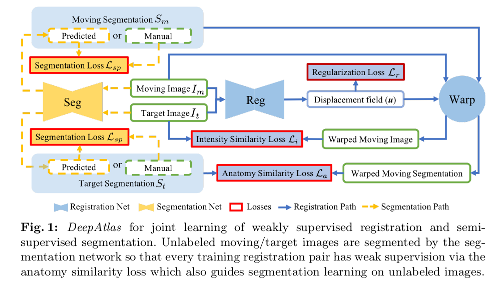They use the Balakrishnan et al. design for the registration network and a customized U-Net for segmentation.

Their approach is based on 2 parts:

• Weakly-supervised registration learning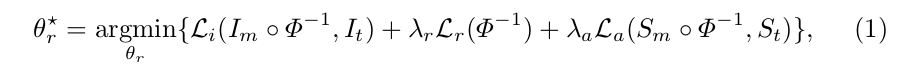where $$\Phi$$ is the spatial transformation applied to the moving image; $$I$$ are the raw image data; and $$S$$ are the label maps.

They estimate missing moving or target segmentations via the segmentation network so that every training registration pair has weak supervision via the anatomy similarity loss.

• Semi-supervised segmentation learning. In addition to the typical supervised segmentation loss (i.e. given a manual segmentation) $$\mathcal{L}_{sp}$$, the anatomy similarity loss term also drives the segmentation.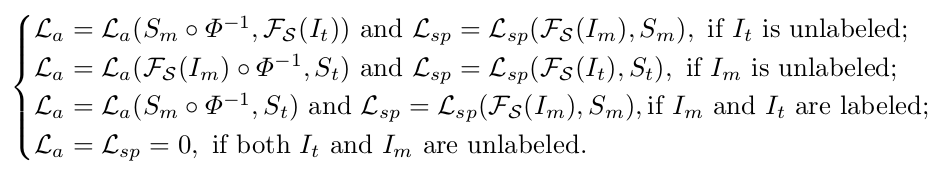where $$\mathcal{F}_{\mathcal{S}}$$ is the segmentation network.

• $$\mathcal{L}_{a}$$ teaches $$\mathcal{F}_{\mathcal{S}}$$ to segment an unlabeled image such that the predicted segmentation matches the manual segmentation of a labeled image via $$\mathcal{F}_{\mathcal{R}}$$.

• $$\mathcal{L}_{a}$$ does not train the segmentation network when both images are labeled.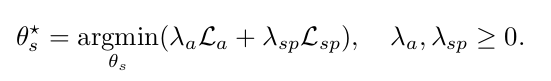The total loss is the weighted sum of the

• The registration regularity loss $$\mathcal{L}_{r}$$ (smoothness)
• The image similarity loss $$\mathcal{L}_{i}$$ (intensity similarity)
• The anatomy loss $$\mathcal{L}_{a}$$ (includes three terms: one for the fixed vs. warped moving image similarities; one for the registration field; and another term for the segmentation maps 1 -an early version of the reference was reviewed here.
• The supervised segmentation loss $$\mathcal{L}_{sp}$$

$$\{ \mathcal{L}_{r}, \mathcal{L}_{i}, \mathcal{L}_{a} \}$$ drive the weakly supervised learning of registration; $$\{ \mathcal{L}_{a}, \mathcal{L}_{sp} \}$$ drive the semi-supervised learning of segmentation.

They alternately train one of the two networks while keeping the other fixed. Since it is difficult to jointly train from scratch with unlabeled images, both networks are pretrained independently.

They use two networks independently trained in a supervised fashion as baselines.

## Data

• Knee MRI experiment: Osteoarthritis Initiative (OAI) dataset. Segmented tissue includes femur and fibula, as well as femoral and tibial cartilage.
• Brain MRI experiment: MindBoogle101 dataset 2 with parcellations of 32 cortical regions.

Used fewer images labeled than images available.

# Results

Accuracy reports:

• Segmentation network: Dice overlap scores between predictions and the manual segmentations.
• Registration network: Dice overlap scores between the warped moving segmentations and the target segmentations.

Two variants:

• DeepAtlas (DA): the joint model is initialized with the trained baselines.
• Semi-DeepAtlas (Semi-DA): a network is trained with the other network held fixed.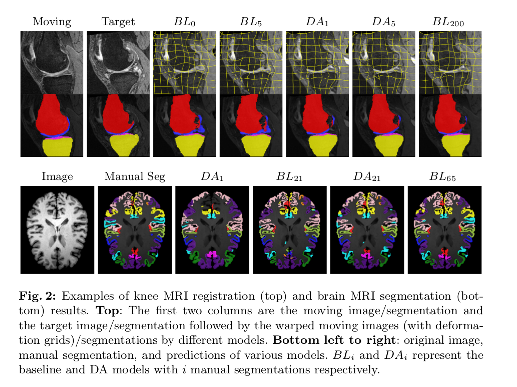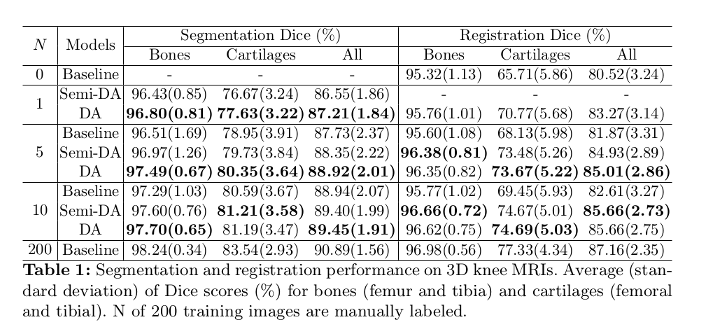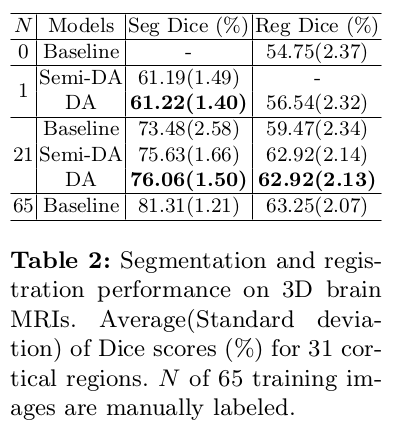# Conclusions

Their approach shows large improvements over separately learned networks.

When only given one manual segmentation, their method provides one-shot segmentation learning and greatly improves registration: one network can benefit from imperfect supervision on unlabeled data provided by the other network.

1. Guha Balakrishnan and Amy Zhao and Mert R. Sabuncu and John Guttag and Adrian V. Dalca. VoxelMorph: A Learning Framework for Deformable Medical Image Registration. Transactions on Medical Imaging. Accepted. 2019.

2. Arno Klein and Jason Tourville. 101 labeled brain images and a consistent human cortical labeling protocol. Frontiers in Neuroscience 6, 171 (2012).